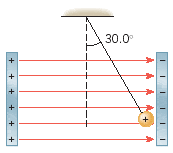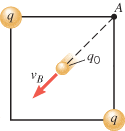# Electric field in plate capacitors

## Homework Statement

A small plastic ball of mass 5.54 x 10-3 kg and charge +0.174C is suspended from an insulating thread and hangs between the plates of a capacitor (see the drawing). The ball is in equilibrium, with the thread making an angle of 30.0o with respect to the vertical. The area of each plate is 0.02030 m^2. What is the magnitude of the charge on each plate?## Homework Equations

Electric Field = Force/test charge Electric field in plate capacitor is sigma/permitivity of space

## The Attempt at a Solution

So....
Tsin30 = mgcos30 becuz in equilibrium
T = 0.0940 which the Force then divide that by test charge gives you the electric field in that area (plate capacitor).

that quotient = charge on plate/epsilon naught * (area of plate) = my answer but its not right. Acutal answer is 3.24 x 10^-8 C

I know I am being greedy but there is another problem I need solved but will have to wait all weekend. I have a poor physics background and I am taking the mcat so any input would be very gracious.

Question: Two identical point charges (q = +9.10 x 10-6 C) are fixed at opposite corners of a square whose sides have a length of 0.460 m. A test charge (q0 = -5.30 x 10-8 C), with a mass of 7.10 x 10-8 kg, is released from rest at one of the corners of the square. Determine the speed of the test charge when it reaches the center of the squarei got an answer of -612 m/s?

Liquidxlax
are you taking into account the force of 1 plate on the ball or both? one plate pushes the other pulls

the second question you should draw a force diagram and use coulomb's law to find force and then acceleration

cupid.callin
For 1st
Draw FBD including firce due to both plates, weight, and tension,

Find X and Y component of tension and use the info that ball is in equilibrium

cupid.callin
second,
use energy conservation